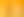How Big?

# Stages1. It's Estimation Time

EXPLORE

Suggested Learning Intentions

• To estimate, measure, and compare different lengths, masses, capacities, and temperatures of common items
• To accurately measure using scaled instruments

Sample Success Criteria

• I can estimate the size of a range of dimensions
• I can measure using a range of units
• I can compare different dimensions of two or more common shapes or objects
• I can explain my thinking using a range of manipulatives
EXPLORE

2. Uncle Jack's Land

EXPLORE

Suggested Learning Intentions

• To compare and contrast perimeter and area
• To explain how to convert between units of linear measurement
• To formulate the rules for areas of two-dimensional shapes
• Students explore and define equable shapes

Sample Success Criteria

• I can explain the difference between perimeter and area
• I can convert between different units of measurement
• I can calculate the area of two-dimensional shapes
• I can define equable shapes
• I can use manipulatives to model and explain my thinking
EXPLORE

3. Do We Have Enough Space?

EXPLORE

Suggested Learning Intentions

• To devise the formulas for area of two-dimensional shapes and to make connections to real-life problem applications of these rules
• To solve problems involving the comparisons of lengths and area using appropriate units

Sample Success Criteria

• I can calculate perimeter and area
• I can solve real-world problems using my knowledge of two-dimensional shapes
• I can model my solutions using a range of manipulatives
EXPLORE

4. Who is Right?

EXPLORE

Suggested Learning Intentions

• To articulate the formulae for areas of two-dimensional shapes
• To explain how to convert between units of linear measurement

Sample Success Criteria

• I can convert between different units of measurement
• I can describe the formulae to calculate areas of two-dimensional shapes
• I can calculate the area of two-dimensional shapes
• I can use concrete materials to model my thinking and justify my solutions
EXPLORE

5. How Big is That Balloon?

EXPLORE

Suggested Learning Intentions

• To compare different lengths, masses, capacities, and temperatures
• To convert between a range of units
• To theorise the volume of a sphere

Sample Success Criteria

• I can compare different measurements
• I can convert between different units of measure
• I can calculate the volume of a sphere
• I can use manipulatives to model and demonstrate my thinking
EXPLORE